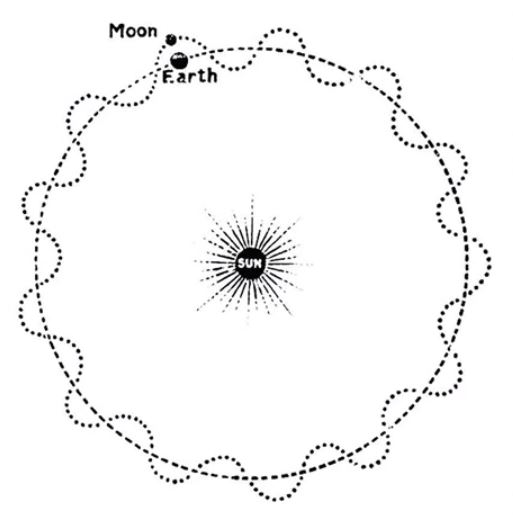# Why Does The Moon Orbit The Earth? [And Not The Sun!]

The Moon is Earth’s only Natural Permanent Satellite, but how did it get there and why does it even orbit around the Earth to begin with? I decided to do some research, here is what I’ve been able to find.

So, why does the Moon orbit the Earth? This is due to what is known as the Hill Sphere. This is a a volume of space where the Earth’s gravity dominates and is able to beat out gravity from something much larger and further away (The Sun). The result is that the Sun pulls on the Earth and the Moon together.

The Sun exerts exerts almost double the amount of gravitational pull on the Moon than does the Earth. Newton’s Law of Universal Gravitation tell us. However, the Moon orbits the Earth but how and why does this actually happen? Let us take a closer look.

## Why Does The Moon Orbit The Earth?

The Moon and the Sun appear to be roughly the the same size in the sky. Despite the fact that the Moon is 400 times smaller than the Sun, it’s also about 400 times closer to Earth than the Sun which causes this effect. It is also why we experience Lunar Eclipses and interesting views of the Moons Phases.

The Sun is massive; making up 99.8% of all the mass in the Solar System. It comes as no surprise to hear that we could line up 109 Earths across its face because it is 330,000x larger!

If we were to use Newton’s proven Law of Gravitation on the Earth, Moon, and the Sun, we discover that the Sun exerts double the gravitational force on the moon than what Earth does.

This can come as quite a surprise and often causes confusion. If the Sun is so massive, and has such a large gravitational pull, can the Moon really orbit the Earth?

Absolutely; and we know through observation, calculation and travelling into Space that the Moon does in fact orbit the Earth. The fact that the Sun exerts higher gravitational pull does not actually matter. Here’s why.

Around everything in Space, the Planets, Moons, Stars – there exists a volume where the gravity of the object in the middle overcomes the gravity from something farther away, even if it is more massive. This is called the Hill sphere.

## What is the Hill Sphere?

When three celestial bodies interact through gravity, their motion is dependent on their position against the Hill Sphere of each.

A body’s Hill Sphere is a region where the gravitational influence of that body is of greater importance than the influence of other (often larger) bodies nearby. It is determined by the independent masses of the different bodies, the semi-major axis and the eccentricity of orbit (i.e. how far the orbit is from perfectly circular).

The Hill sphere around the Earth has a radius of about one and a half million kilometers, which is more than enough to fit the moon’s orbit.

If the Moon was further away, or if the Earth and the Moon were any closer to the Sun, then the Moon would not orbit us here on Earth.

The important thing to consider is that inside the Hill sphere, the Earth’s gravity dominates, and
the Sun pulls on Earth and the Moon together (almost as one entity together).

So in actuality, The moon does orbit the Sun indirectly, it just orbits the Earth more.

## What Does The Moons Orbit Look Like?

The path the Moon takes as it travels with Earth around the Sun is an interesting one. You can actually imagine how it works through the following analogy:

If you imagine two cars (one the Earth, the other the Moon) racing around a circular track. One of the cars (Moon) speeds up to pass the other (Earth), which is moving at a constant speed. The outer car (Moon) then moves left and gets overtaken by the other car (Earth). Ultimately, the car illustrating the Moon would be making circles around the car illustrating the Earth. When seen from above, both cars (Earth and Moon) are driving forward all the time and the path is convex. From a vantage point, it will look like this:But as the distance to the Moon isn’t much compared to the space between the Earth to the Sun, during the gravitational dance around the Sun, those bumps smooth out.

## Interesting Facts

• It takes the Moon around 27 days to completely orbit the Earth.
• The Moon is in synchronous rotation. This means that it shows the same face towards Earth at all times.
• The Moon orbits Earth anticlockwise and the Earth orbits the Sun anticlockwise,
• The Moon and Earth rotate on their own axes anticlockwise.
• The orbit of the Moon is a nearly a circular Ellipse about the Earth.
• With a lower mass, the Moon is affected by the Earth’s gravity more than the Earth is affected by the Moon.
• One of the Moon’s most important effects on the Earth is its large influence over our Ocean Tides.
• The moon formed around 4.5 billion years ago. This is around 30–50 million years after the creation of the Solar System. It was the result of a massive collision between two planetoids.
• There is a constant and continuous force that maintains the balance of celestial bodies in this universe.
error: Content is protected !!